# ABSTRACT.

To approximate a simple root of a real function f we construct a family of iterative maps, which we call Newton-barycentric functions, and analyse their convergence order. The performance of the resulting methods is illustrated by means of numerical examples.

Keywords:
order of convergence; Newton's method; Newton-barycentric map; nonlinear equations

# RESUMO.

Com o fim de aproximar uma raiz simples de uma função real f, constrói-se uma família de aplicações iteradoras, que se designam funções Newton-baricêntricas, e analisa-se a sua ordem de convergência. O desempenho dos métodos computacionais resultantes é ilustrado através de exemplos numéricos.

Palavras-chave:
Ordem de convergência; método de Newton; função Newton-baricêntrica; equações não-lineares

# 1 INTRODUCTION

The classical Newton's iterative scheme for approximating the roots of an equation has been generalized by many authors in order to define iterative maps going from cubical to arbitrary orders of convergence (see for instance 11 L. Collatz. Functional Analysis and Numerical Mathematics. Academic Press, New York (1966).), (22 A. Cordero & J. Torregrosa. Variants of Newton's method using fifth-order quadrature formulas.Appl. Math. Comput., 190 (2007), 686-698.), (33 M. M. Graça & P. M. Lima. Root finding by high order iterative methods based on quadratures.Appl. Math. Comput., 264 (2015), 466-482.), (55 Y. Ham, C. Chun & S.-G. Lee. Some higher-order modifications of Newton's method for solving nonlinear equations. J. Comp. Appl. Math., 222 (2008), 477-486.), (66 A. S. Householder. The Numerical Treatment of a Single Nonlinear Equation. McGraw -Hill, New York (1970).), (88 G. Labelle. On extensions of the Newton-Raphson iterative scheme to arbitrary orders. Disc. Math Th. Comput Sc. (DMTCS), proc. AN, 845-856, Nancy, France (2010).), (99 W. C. Rheinboldt. Methods for Solving Systems of Nonlinear Equations. 2nd Ed., SIAM, Philadelphia (1998).), (1010 P. Sebah & X. Gourdon. Newton's method and high order iterations, 2001. Available from: http://numbers.computation.free.fr/Constants/constants.html.
http://numbers.computation.free.fr/Const...
), (1111 G. Fernandez-Torres. Derivative free iterative methods with memory of arbitrary high convergence order. Numer. Alg., 67 (2014), 565-580.), (1212 J. F. Traub. Iterative Methods for the Solution of Equations. Prentice-Hall, Englewood Cliffs (1964).), (1313 S. Weerakoon & G. I. Fernando. A variant of Newton's method with accelerated third-order convergence. App. Math. Lett., 13 (2000), 87-93., and references therein). In particular we mention the third-order method introduced in 1313 S. Weerakoon & G. I. Fernando. A variant of Newton's method with accelerated third-order convergence. App. Math. Lett., 13 (2000), 87-93. and a family of high-order methods based on quadrature rules, recently described in 33 M. M. Graça & P. M. Lima. Root finding by high order iterative methods based on quadratures.Appl. Math. Comput., 264 (2015), 466-482..

In that work the authors have introduced a recursive algorithm for constructing iterative maps. The first iterative function of this family is the classical Newton's method, which can be seen as the result of applying the rectangles rule to approximate a certain integral. As it is well-known, Newton's method has, in general, second order of convergence (when applied to the computation of simple roots). In the referred work 33 M. M. Graça & P. M. Lima. Root finding by high order iterative methods based on quadratures.Appl. Math. Comput., 264 (2015), 466-482., the authors have shown that iterative maps of arbitrarily high order can be obtained if quadrature rules of higher degree are used (instead of the rectangles rule) to compute the mentioned integral. The properties of these methods were analysed and their convergence has been illustrated by several numerical examples.

In the present work we also construct a family of high order iterative maps, starting with the Newton's method, but we follow a different approach. All the iterative maps t to be considered have the common structure t(x) = x - [ϕ(x)]-1 f(x), where f is a real function with a simple zero at z. The function ϕ will be called a model function. This model function depends on f and on another function h which we name step function.

The family of iterative maps to be discussed is constructed by choosing a certain model function ϕ. We remark that our approach does not follow the traditional path for generating iterative maps by direct or inverse hyperosculatory interpolation (see, for instance 1212 J. F. Traub. Iterative Methods for the Solution of Equations. Prentice-Hall, Englewood Cliffs (1964).) or Taylor expansions around a zero of f (see for instance 1010 P. Sebah & X. Gourdon. Newton's method and high order iterations, 2001. Available from: http://numbers.computation.free.fr/Constants/constants.html.
http://numbers.computation.free.fr/Const...
).

Given a real -valued function f defined on an open set D ⊂ ℝ, we assume that f is sufficiently smooth in a neighbourhood of a simple zero z of f. In what follows we show how to construct a family of iterative maps t generating a sequence xi + 1 = t(xi ), i = 0, 1, ..., converging locally to z.

Maps of higher order of convergence can produce accurate approximations with a smaller number of iterations. However, in general, they have expressions of increasing complexity, and so augmenting its computational cost. In this paper we show that at least for some Newton-barycentric methods the gain in accuracy compensates the computational cost. Like other root-finding methods these iterative maps can be easily extended to the case of multivariate functions. Numerical experiments have been carried out and the results are promising. The corresponding theory is still under construction.

The paper is organized as follows. In Section 2 we introduce the notion of a model function ϕ and prove that the iterative map t(x) = x - [ϕ(x)]-1 f(x) has a certain order of convergence (see Proposition 2.1). This is the main idea for the construction of recursive families of iterative functions.

In Section 3 we describe in detail one of these families, which we call the Newton-barycentric iterative maps. We begin by specifying the choice of the model function in the case of this family (Subsection 3.1). Then we describe the algorithm for obtaining iterative maps, based on this model function and we give the formulae of some of these maps (Subsection 3.2). In Section 4 we analyse the application of some Newton-barycentric formulas to some numerical examples. We finish in Section 5 with some conclusions.

# 2 RECURSIVE FAMILIES OF ITERATIVE MAPS

In this section we describe a procedure for constructing recursive families of higher order iterative methods.

Proposition 2.1. Let z be a simple zero of a function f : D ⊂ ℝ ⟼ ℝ and ϕ a sufficiently smooth function in a neighbourhood of z, such that its derivatives ϕ ( i ) satisfy the j + 1 equalities

(2.1)for j ≥ 0 a fixed integer. Then, for any initial value x 0 sufficiently close to z, the iterative process xk + 1 = t(xk ), k = 0, 1, ..., with

(2.2)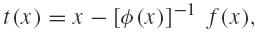converges to z and its order of convergence is at least j + 2.

Proof. From (2.2) it is obvious that the zero z of f is a fixed point of the map t (that is, t(z) = z). Let us consider the function Δt defined by

Δt(x) = t(x) - x.

Note that its derivatives are

Δ(1) t(x) = t (1)(x) - 1, and Δ( i ) t(x) = t ( i )(x), for i ≥ 2.

We now use induction on j to prove that the hypotheses in (2.1) imply that Δt(z) = 0, Δ(1) t(z) = -1, Δ( i ) t(z) = 0, for j ≥ 2, and consequently t has the referred order of convergence.

Let j = 0. Rewriting (2.2) as

(2.3)and applying the derivative operator to this equation, we have

(2.4)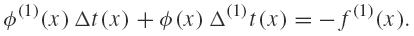Since Δt(z) = 0, and ϕ satisfies (2.1) with i = 0, it follows that f (1)(z(1) t(z) = -f (1)(z). As z is a simple zero for f, then

Δ(1) t(z) = -1 ⇔ t (1)(z) = 0,

which means that the iterative process generated by t has local order of convergence p at least 2. That is, p ≥ j + 2.

Let j = 1. Differentiating (2.4), we get

ϕ(2)(xt(x) + 2ϕ(1)(x(1) t(x) + ϕ(x(2) t(x) = f (2)(x).

Since Δt(z) = 0 and Δ(1) t(z) = -1, we obtainTherefore Δ(2) t(z) = t (2)(z) = 0, and so the iterative process has local order of convergence at least 3 = j + 2.

For an integer m ≥ 2, assume thatand

(2.5)Let us show that Δ( m + 1) t(z) = t ( m )(z) = 0. From (2.3) and the Leibniz's rule for the derivatives of the product, we have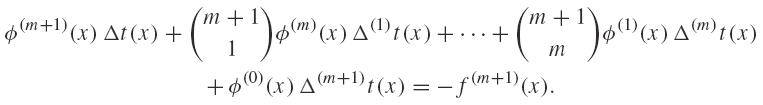Thus, by the induction hypotheses, we obtainHence the iterative map tm + 1 has local order of convergence p ≥ m + 2 and the proof is complete.

Remark 2.1. The well-known result on the local order of convergence of the Newton's map t(x) = x - [f (1)(x)]-1 f(x) follows immediately from Proposition 2.1. It is enough to see that (2.1) is verified for j = 0, i.e. ϕ(0)(z) = f (1)(z), and so t has local order of convergence at least 2.

With the aim of analysing in detail the process of creating iterative maps, proposed in Proposition 2.1, we will now introduce the definitions of model function and step function.

Definition 2.1. Let z be a simple zero of a function f : D ⊂ ℝ ⟼ ℝ, h and ϕ sufficiently smooth functions in a neighbourhood of z, and j ≥ 0 a fixed integer.

• A function ϕ is called a model function of degree j, at x = z, if it satisfies the j + 1 conditions (2.1), but does not satisfy a similar condition for i = j + 1.

• A function h is called a step function of degree j at x = z (or simply a step function) if it satisfies the following j + 1 equalities:

(2.6)but does not satisfy the condition corresponding to i = j + 1.

Example 2.1. Under the conditions of Definition 2.1, set

(2.7)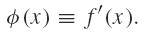Then we have ϕ'(z) = f''(z) but ϕ''(z) = f'''(z) ≠ f'''(z)/2. Therefore, ϕ defined by (2.7) is a model function of degree 0.

In the same way, let

(2.8)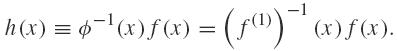In this case, h(z) = f(z)/f'(z) = 0, but h'(z) = 1 - f(z)f"(z)/(f(z))2 = 1 ≠ -1. Hence h defined by (2.8) is a step function of degree 0.

The above defined model function ϕ and step function h can be used to define the map t(x) = x - [f (1)(x)]-1 f(x), which coincides with the Newton's method (see Remark 2.1).

# 3 THE NEWTON-BARYCENTRIC MAPS

We now consider a family of iterative maps, based on the model function ϕk , defined as follows: ϕk is the inner product of a constant vectorial function Uk and a function Vk depending only on the first derivative f (1) evaluated at x + i h(x), for i = 0, ..., k. Namely, we take

(3.1)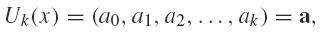where the choice of a will be discussed below, and

(3.2)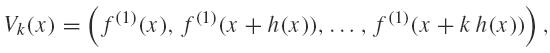where h is a step function of degree k. If one proves that ϕk = 〈a, Vk (x)〉 is a model function of degree k then, by Proposition 2.1, the respective process tk (x) = x - [ϕk (x)]-1 f(x) has order of convergence at least k + 2.

## 3.1 Choice of the model function

The next proposition shows that ϕk is a model function of degree k if and only if Uk (x) = a is the unique solution of a non homogeneous linear system. Moreover, this solution represents the barycentric coordinates of ϕk in a basis defined by the components of Vk . The name Newton-barycentric maps reflects this property, as well as the fact that the recursive process starts with the Newton's method.

Proposition 3.1. Let f be a function satisfying the hypotheses of Proposition 2.1, h a step function of degree k, k ≥ 0 a fixed integer and ϕk = 〈Uk, Vk 〉, with Uk and Vk defined by (3.1) and (3.2). That is,

(3.3)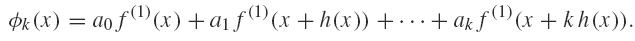Then, the derivative of order k of Vk, evaluated at x = z, is Vk (k)(z) = DkRk, where Dk and Rk are the following (k + 1)×(k + 1) matrices

Dk = diag(f (1)(z), f (2)(z), ..., f ( k + 1)(z)),

and

(3.4)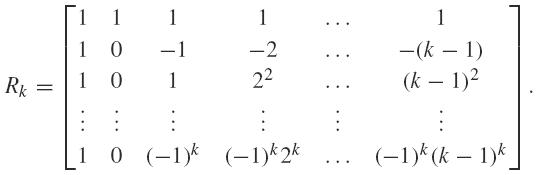Furthermore,

(i) The function ϕk is a model function of degree k if and only if

Uk = a = (a 0, a 1, a 2, ..., ak )

is the (unique) solution of the linear system

(3.5)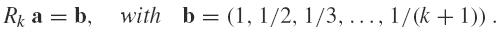In particular, this solution satisfies the equality

(3.6)(ii) If f ( i )(z) ≠ 0 for i = 1, ..., k, then the function Uk = a represents the (normalized) barycentric coordinates of the model function ϕk relative to the basis,

𝒱k = {f (1)(x), f (1)(x + h(x)), ..., f (1)(x + kh(x))}.

Moreover, the iterative process generated by tk (x) = x - [ϕk (x)]-1 f(x) has order of convergence at least k + 2.

Proof. For i = 0, 1, ..., k the derivatives of order i of Vk , evaluated at x = z, are:

Vk (0) = f (1)(z) (1, 1, 1, 1, 1, ..., 1)

Vk (1) = f (2)(z) (1, 0, -1, -2, -3, ..., -(k - 1))

Vk (2) = f (3)(z) (1, 0, 1, 22, 32, ..., (k - 1)2)

.

.

.

Vk (k) = f ( k + 1)(z) (1, 0, (-1)k , (-1)k 2k , (-1)k 3k , ..., (-1)k (k - 1)k ).

So, the equalities (3.5) hold.

For (i), since ϕk = 〈a, Vk (x)〉, it is straightforward to verify that the conditions (2.1) for ϕk to be a model function are equivalent to the system Rk a = b. So, Uk = a must be a solution of this system. As Rk is nonsingular, this is the unique solution. Furthermore, since z is a simple zero of f, the equality (3.6) holds because it is just the first equation of the system Rk a = b.

For (ii), we need to show that for α = (α0, α1, ..., αk ) ∈ ℝk + 1, such that

(3.7)the only solution is α = 0. Differentiating (3.7) and evaluating at x = z, we obtain the homogeneous linear system

diag(f (1)(z), f (2)(z), ..., f ( k + 1)(z))Rk α = 0,

which admits only the solution α = 0 since both the diagonal matrix and Rk are nonsingular.

The last assertion follows from Proposition 2.1 since by item (i) ϕk is a model function of degree k. □

The expressions for the first five barycentric maps are shown in Table 1.

Table 1:
First five barycentric type maps.

## 3.2 Construction of the recursive family of iterative maps

We recall that a model function ϕ depends on a certain step function h. Now, for each model function ϕj included in the definition of the map tj = x - [ϕj (x)]-1 f(x), we use a step function which is defined recursively by hj (x) = tj - 1(x) - x. The starter t 0 will be taken to be the Newton's map t 0(x) = x - [f (1)(x)]-1 f(x). The next proposition shows that the iterative map tm , defined recursively in (3.8), has local order of convergence at least m + 2.

Proposition 3.2. Let z be a simple zero of a given function f and t 0 the Newton's map

t0(x) = x - [f (1)(x)]-1 f(x).

For a given natural number m ≥ 1, define recursively the step function hm and the iterative map tm by

(3.8)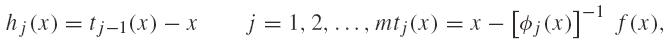where ϕj is constructed using hj as step function and ϕj is a barycentric-type map, given by (3.3)-(3.4). Then, the map tm has local order of convergence at least m + 2.

Proof. It is only necessary to prove that each function hj is a step function of degree j and the statement follows from Proposition 3.1.

Let us apply induction on the integer m. For m = 1, we have h 1(x) = t 0(x) - x and so h 1(z) = 0 and h 1 (1)(z) = -1.

Let m ≥ 1 be an integer. As hm (0)(z) = 0, hm (1)(z) = -1 and for any integer i such that 2 ≤ i ≤ m, we have hm ( i )(z) = 0, and so hm is a step function of degree m. □

The Newton-barycentric maps tk of arbitrary degree k are thus completely defined by Proposition 3.2. Let us remark that the same procedure to construct recursive families of iterative methods can be applied using other types of model and step functions. Moreover the idea of this method is easily extendable to the case of multivariate functions. Multidimensional analogs of the Newton-barycentric maps have been implemented and applied to the solution of systems of nonlinear equations and the numerical results obtained so far are promising.

# 4 NUMERICAL EXAMPLES

Besides the theoretical interest of this new family of iterative functions, some of the above described methods are of practical interest. Though they are not optimal in the sense of the Kung and Traub's conjecture 77 H. T. Kung & J. F. Traub. Optimal order of one-point and multipoint iteration. J. Assot. Comput. Math., 21 (1974), 634-651., they are more efficient than the Newton's method, in the sense that with the same number of function evaluations a more accurate result can be produced. Concerning for example the Newton's method (iterative function t 0) and the method with the iterative function t 1, we know that the first one requires 2 function evaluations at each iteration, while the second one requires 3. This means that two iterations of the second method require as many function evalutions (6) as three iterations of the first one. However the result produced by two iterations of the second method is in general much more accurate than the one produced by 3 iterations of the Newton's method. This happens, because the second method has at least convergence order three, and it follows that t 1t 1 (the composition of t 1 with itself) has at least convergence order 9; on the other hand, since the Newton method has in general convergence order 2, the method t 0t 0t 0 has just convergence order 8. Concerning the method t 2 (fourth order of convergence) each iteration requires 5 function evaluations; however as we shall see in the examples below, one single iteration of this method is often sufficient to obtain a result with an error less than 10-10 (which can only be obtained with three iterates of the Newton's method).

Let us present some numerical results that illustrate the above properties. In all the cases we have applied the methods with the iterative functions t 0, t 1 and t 2, starting with a certain initial approximation x 0 and performing enough iterations to obtain an approximation with absolute error less than10-10. Then we compare the error of the last approximation, the number of iterations and the number of function evaluations by each method. Moreover we have obtained computational estimates of the convergence order, which confirm the theoretical predictions. All the computations were carried out in a personal computer using Mathematica1414 S. Wolfram. The Mathematica Book. Wolfram Media, fifth ed., (2003).. The results are displayed in Tables 2, 3, 4 and they illustrate the advantage of using the methods with the iterative functions t 1 and t 2 for approximating the roots of real functions.

Table 2:
f(x) = x3 + 4x 2 - 10, the initial approximation is x 0 = 1, the solution is z = 1.3652300134141, with 12 significant digits (see this example in 1313 S. Weerakoon & G. I. Fernando. A variant of Newton's method with accelerated third-order convergence. App. Math. Lett., 13 (2000), 87-93.).

Table 3:
f(x) = cos(x) - x, the initial approximation is x0 = 0.1, the solution is z = 0.739085133215, with 12 significant digits.

Table 4:
f(x) = tanh(x - 1), the initial approximation is x 0 = 0, the exact solution is z = 1.

# 5 CONCLUSIONS

In this work we have introduced a family of high order iterative methods for the numerical solution of nonlinear equations. This family starts with the Newton's method as the basis of the recurrence process. Then each member of the family is build by a well defined procedure, forming a sequence of increasing convergence order.

The numerical examples presented in Section 4 demonstrate that the first iterative functions of these family (in particular t 1 and t 2) offer a good alternative to the Newton's method, taking account their accuracy and number of function evaluations. We remark that these methods can be easily combined with algorithms for the separation of real roots, such as recently described in 44 M. M. Graça. Maps for global separation of roots. Electronic Transactions on Numerical Analysis, 45 (2016), 241-256., providing an effective tool for detection and computation of real roots.

# ACKNOWLEDGMENTS

The authors would like to thank the anonymous referees for helpful comments and suggestions.

# REFERENCES

• 1
L. Collatz. Functional Analysis and Numerical Mathematics. Academic Press, New York (1966).
• 2
A. Cordero & J. Torregrosa. Variants of Newton's method using fifth-order quadrature formulas.Appl. Math. Comput., 190 (2007), 686-698.
• 3
M. M. Graça & P. M. Lima. Root finding by high order iterative methods based on quadratures.Appl. Math. Comput., 264 (2015), 466-482.
• 4
M. M. Graça. Maps for global separation of roots. Electronic Transactions on Numerical Analysis, 45 (2016), 241-256.
• 5
Y. Ham, C. Chun & S.-G. Lee. Some higher-order modifications of Newton's method for solving nonlinear equations. J. Comp. Appl. Math., 222 (2008), 477-486.
• 6
A. S. Householder. The Numerical Treatment of a Single Nonlinear Equation. McGraw -Hill, New York (1970).
• 7
H. T. Kung & J. F. Traub. Optimal order of one-point and multipoint iteration. J. Assot. Comput. Math., 21 (1974), 634-651.
• 8
G. Labelle. On extensions of the Newton-Raphson iterative scheme to arbitrary orders. Disc. Math Th. Comput Sc. (DMTCS), proc. AN, 845-856, Nancy, France (2010).
• 9
W. C. Rheinboldt. Methods for Solving Systems of Nonlinear Equations. 2nd Ed., SIAM, Philadelphia (1998).
• 10
P. Sebah & X. Gourdon. Newton's method and high order iterations, 2001. Available from: http://numbers.computation.free.fr/Constants/constants.html
» http://numbers.computation.free.fr/Constants/constants.html
• 11
G. Fernandez-Torres. Derivative free iterative methods with memory of arbitrary high convergence order. Numer. Alg., 67 (2014), 565-580.
• 12
J. F. Traub. Iterative Methods for the Solution of Equations. Prentice-Hall, Englewood Cliffs (1964).
• 13
S. Weerakoon & G. I. Fernando. A variant of Newton's method with accelerated third-order convergence. App. Math. Lett., 13 (2000), 87-93.
• 14
S. Wolfram. The Mathematica Book. Wolfram Media, fifth ed., (2003).

# Publication Dates

• Publication in this collection
Sep-Dec 2016